Courses
Courses for Kids
Free study material
Free LIVE classes
More

# JEE Important Chapter - Properties of Solids and Liquids

Get interactive courses taught by top teachers## Properties of Solids and Liquids for JEE

Last updated date: 26th May 2023
Total views: 107.7k
Views today: 5.61k

The chapter Properties of solids and liquids is one of the most important chapters for JEE Main. It mainly deals with the behaviour of solids under stress and liquids at rest and motion.

Related to the properties of solid, we learn about types of stresses and strain followed by the types of  modulus of elasticity. The stress-strain curve of a material and Hooke's law is explained in detail in this chapter. Thermal stress and work done in stretching a wire are also discussed in this chapter in detail.

In properties of liquid, Pascal’s law and its applications are studied along with Archimedes's principles and numerical problems related to it. Bernoulli's theorem and its application and problems are very important topics in this chapter. Viscosity and surface tension is also explained in detail in this chapter. We have seen some conversion of solid to liquid examples and also liquid to solid in lower grades. A solid to liquid example of its conversion is the melting of ice.

Let us see what are solids and liquids properties and the important concepts and formulas needed for JEE Main in this chapter properties of solids and liquids with solved examples.

## JEE Main Physics Chapter-wise Solutions 2022-23

 JEE Main Physics Chapter-wise Solutions 1 Units and Measurement 11 Electrostatics 2 Kinematics 12 Current Electricity 3 Laws of Motion 13 Magnetic Effects of Current and Magnetism 4 Work, Energy and Power 14 Electromagnetic Induction and Alternating Currents 5 Rotational Motion 15 Electromagnetic Waves 6 Gravitation 16 Optics 7 Properties of Solids and Liquids 17 Dual Nature of Matter 8 Thermodynamics 18 Atoms and Nuclei 9 Kinetic Theory of Gases 19 Electronic Devices 10 Oscillations and Waves 20 Communication Systems

### Properties of Solids and Liquids - Important Topics

• Elasticity of solids and its elastic behaviour

• Young’s modulus

• Bulk modulus

• Rigidity modulus

• Stress-strain curve

• Pascal’s Law

• Mercurial Barometer

• Archimedes Principle

• Bernoulli’s Therorem

• Application of Bernoulli’s theorem

• Stoke’s Law

• Surface tension and surface energy

### Important Concepts of Properties of Solids and Liquids

 Name of the Concept Key Points of the Concept Elasticity of Solids and its elastic behaviour Some solids can regain its shapee and size after deforming force is removed. This is called elasticity.If the body cannot regain its shape and size, then the body is said to be plastic. The body undergo permanent deformation due to its plastic behaviour.Stress is the restoring force acting per unit area of cross-section of the body. The unit of stress is N/m2. The different types of stress are longitudinal stress, volume stress and tangential stress.$\text{stress}=\dfrac{\text{Force}}{\text{Area}}$The strain is the ratio of change in dimension to the original dimension when the deforming force is applied.The different types of strain are longitudinal strain, volume strain and shearing strain. Young’s Modulus Young’s modulus is the ratio of longitudinal stress to the longitudinal strain.$\text{Young's modulus}=\dfrac{\text{Longitudinal stress}}{\text{longitudinal strai}}$$\text{Young's modulus}=\dfrac{F/A}{\Delta l/l}Longitudinal stress occurs in solids only when the deforming force is applied parallel to the length of the wire.Longitudinal strain is the ratio of change in length to the original length due to longitudinal stress.\text{Longitudinal strain}=\dfrac{\text{change in length}}{\text{original length}}$$\text{Longitudinal strain}=\dfrac{\Delta l}{l}$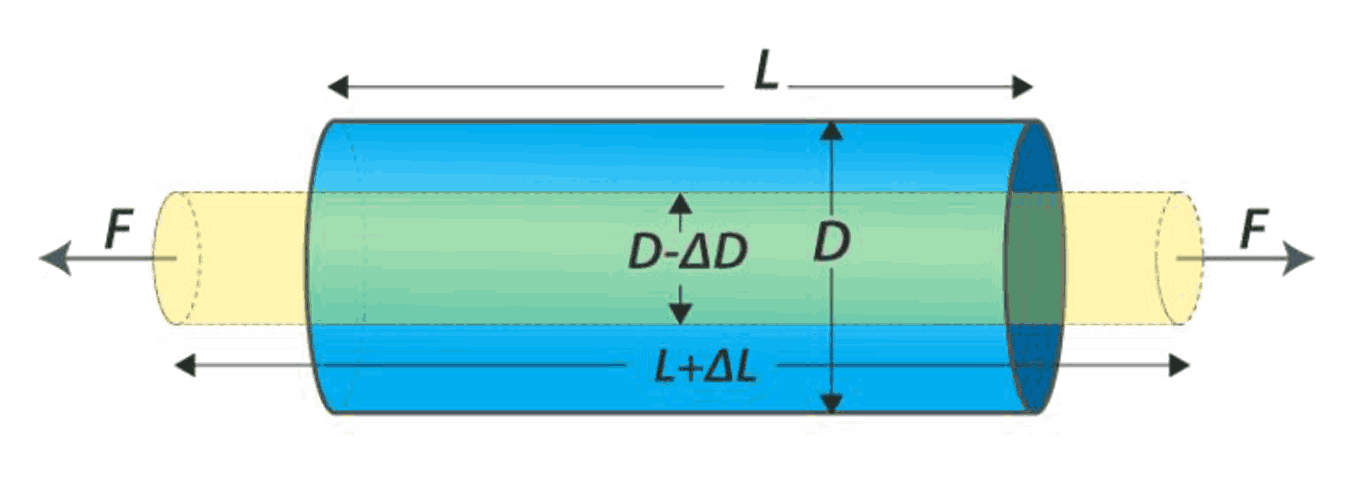Bulk Modulus Bulk modulus is the ratio of normal stress to the volume strain given by the formula,$\text{Bulk modulus}=\dfrac{\text{Normal stress}}{\text{Volumetric strain}}$$\text{Bulk modulus}=\dfrac{F/A}{-\Delta V/V}$$\text{Bulk modulus}=\dfrac{-PV}{\Delta V}$Bulk stress or volume stress can act in solids, liquids and gases and it produces volume strain.Volume strain is the ratio of change in volume to the original volume given by the formula,$\text{Volume strain}=\dfrac{\text{change in volume}}{\text{original volume}}$$\text{Longitudinal strain}=\dfrac{\Delta V}{V}(Image will be uploaded soon) Rigidity modulus Modulus of rigidity is the ratio of tangential stress to the shearing strain given by,\text{Rigidity modulus}=\dfrac{\text{shearing stress}}{\text{shearing strain}}$$\eta=\dfrac{F/A}{\text{x/h}}$Tangential stress or shear stress is developed when the deforming force is applied tangentiallyShearing strain is defined as the angle in radians through which the vertical surface gets turned due to tangential force and its formula is given by,$\text{Shearing strain}=\dfrac{\text{x}}{h}$ The diagram show an example of solid cube undergoing shear stress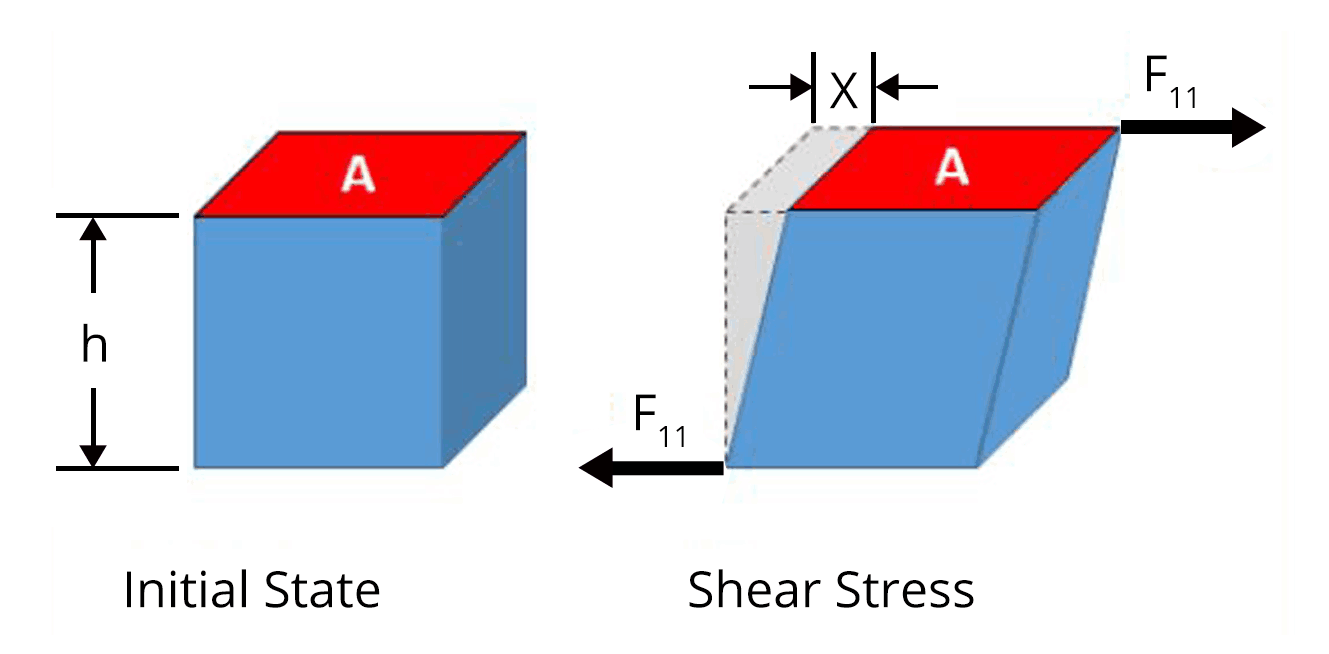Stress-strain curve The behaviour of a material can be studied using stress-strain curve.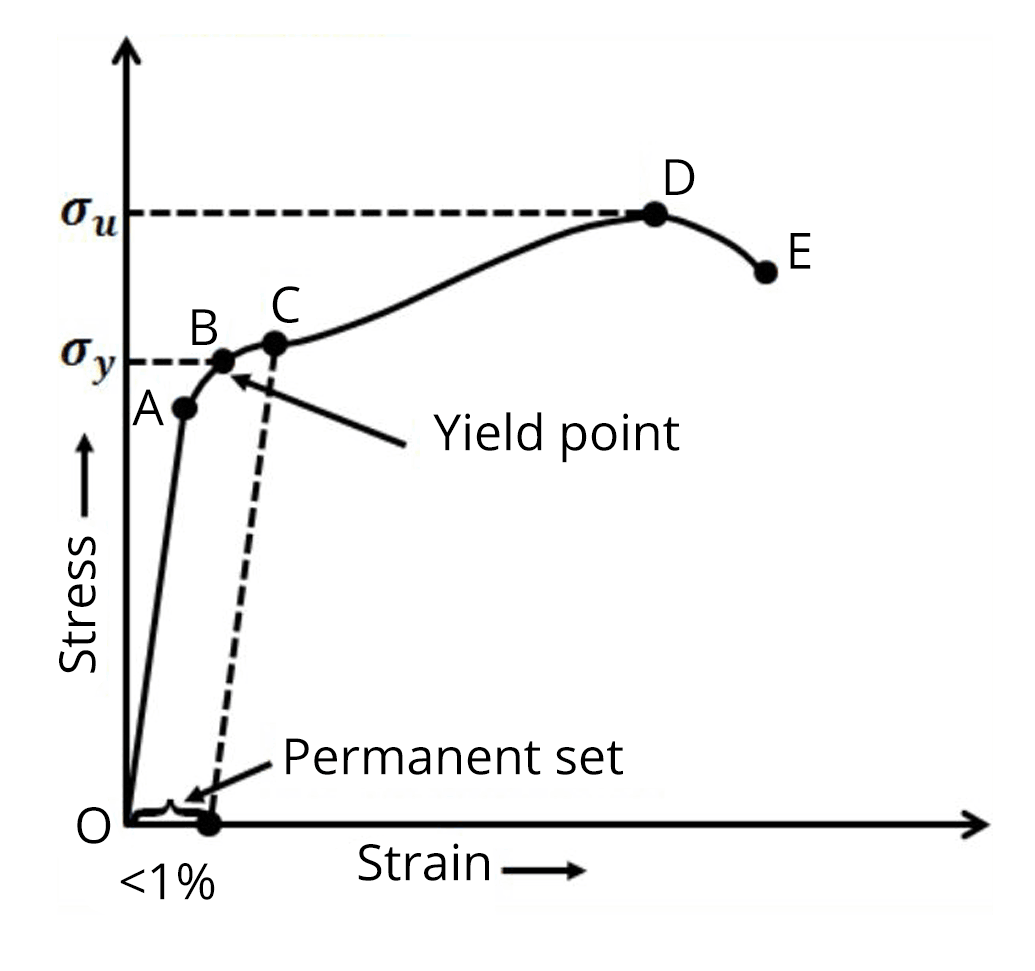The curve obeys Hooke’s law in the region from O to A. According to Hookes law. Stress is proportional to strain.In the stress-strain curve given above, the point A corresponds to proportional limit. The yield point or elastic limit is the point upto which the wire shows elastic behaviour and the point B corresponds to the yield point.The stress corresponding to the yield point is called yield strength.Beyond point B, it loses its elastic behaviour and does not regain its original length even after deforming force is removed.The stress corresponding to point  D is called ultimate strength. Pascal’s Law A change in pressure at any point in a confined incompressible fluid at rest is transmitted undiminished to all points in the fluid.(Image will be uploaded soon)The application of pascal’s law are used in hydraulic lift, hydraulic brakes and press.In hydraulic lift, a small force is converted to a large force having many times the magnitude of the small force. This is a good example of liquid obeying pascal’s law.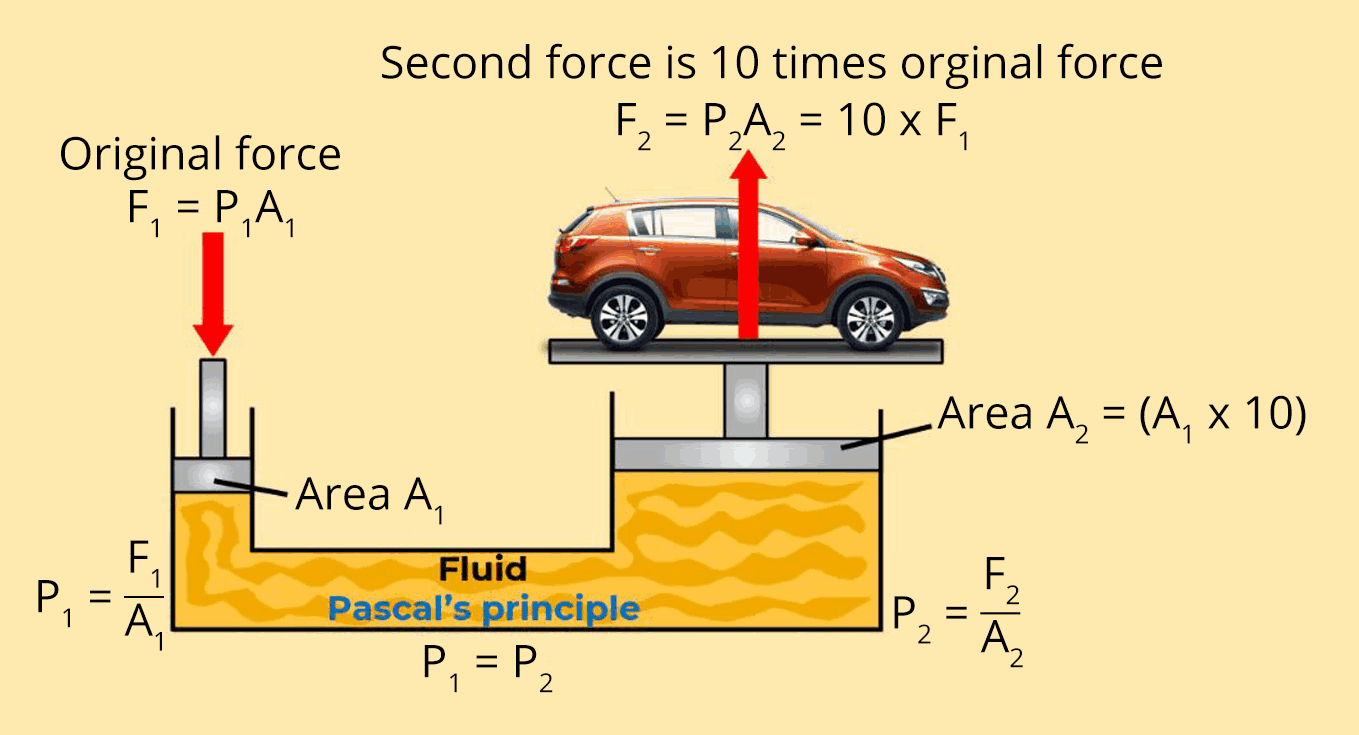Mercurial Barometer Mercurical barometer is used to measure the atmospheric pressure. The fluid pressure corresponds to height of the mercury in the column is equal to the atmospheric pressure.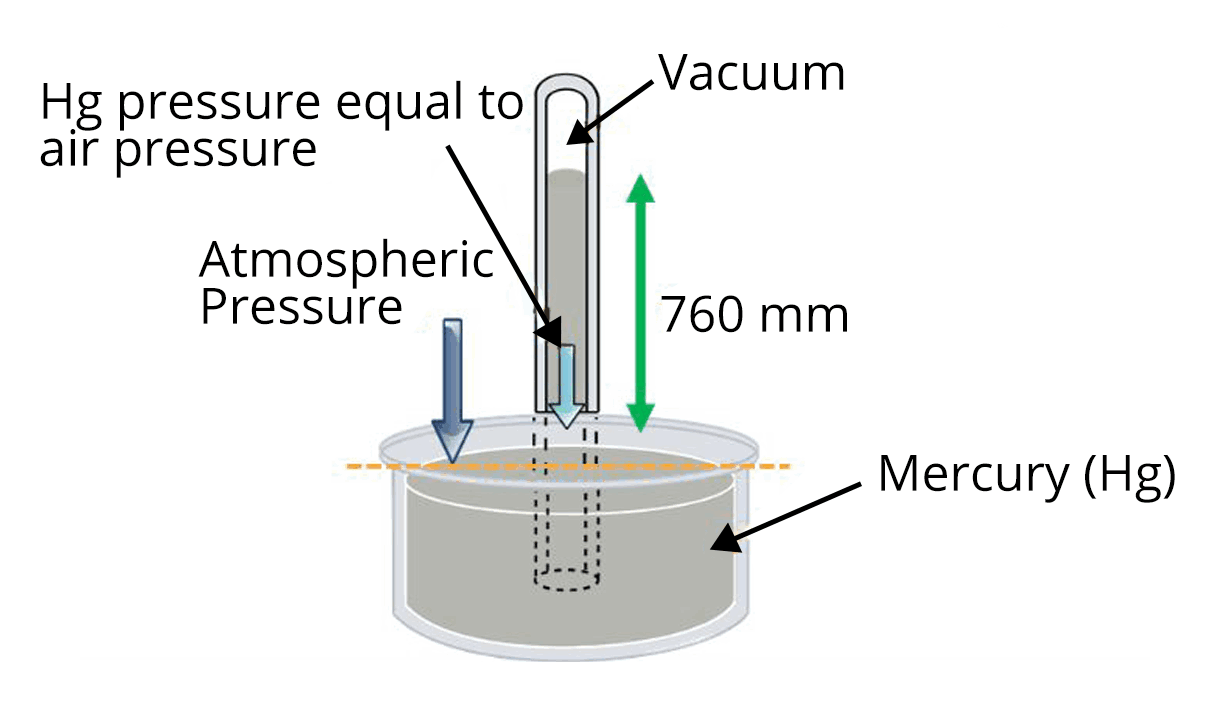Archimedes Principle According to Archimedes principle, whenever a body is fully or partially submerged in liquid, it experiences a net upward force which is equal to the weight of the liquid displaced.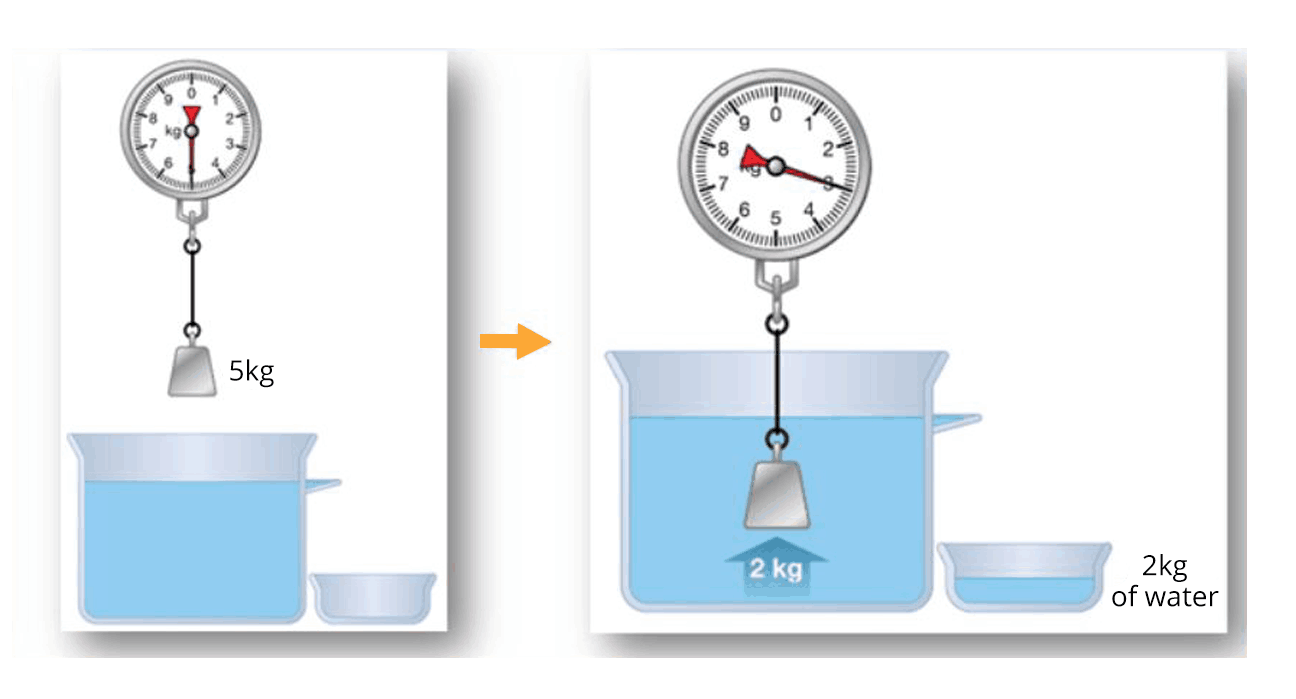The formula to calculate the buoyancy force is given by,$F_B=\rho_l Vg$Where ⍴l is the density of the liquid, V is the volume of the body immersed in the liquid and g is the acceleration due to gravity.A body will float in a liquid if the buoyancy force acting on the body is equal to the weight  of the body. Bernoulli’s Theorem According to Bernoulli’s theorem, the total energy per unit volume of an incompressible fluid always remains constant at any point of the fluid.$P+\rho gh+\dfrac{1}{2}\rho v^2=\text{constant}$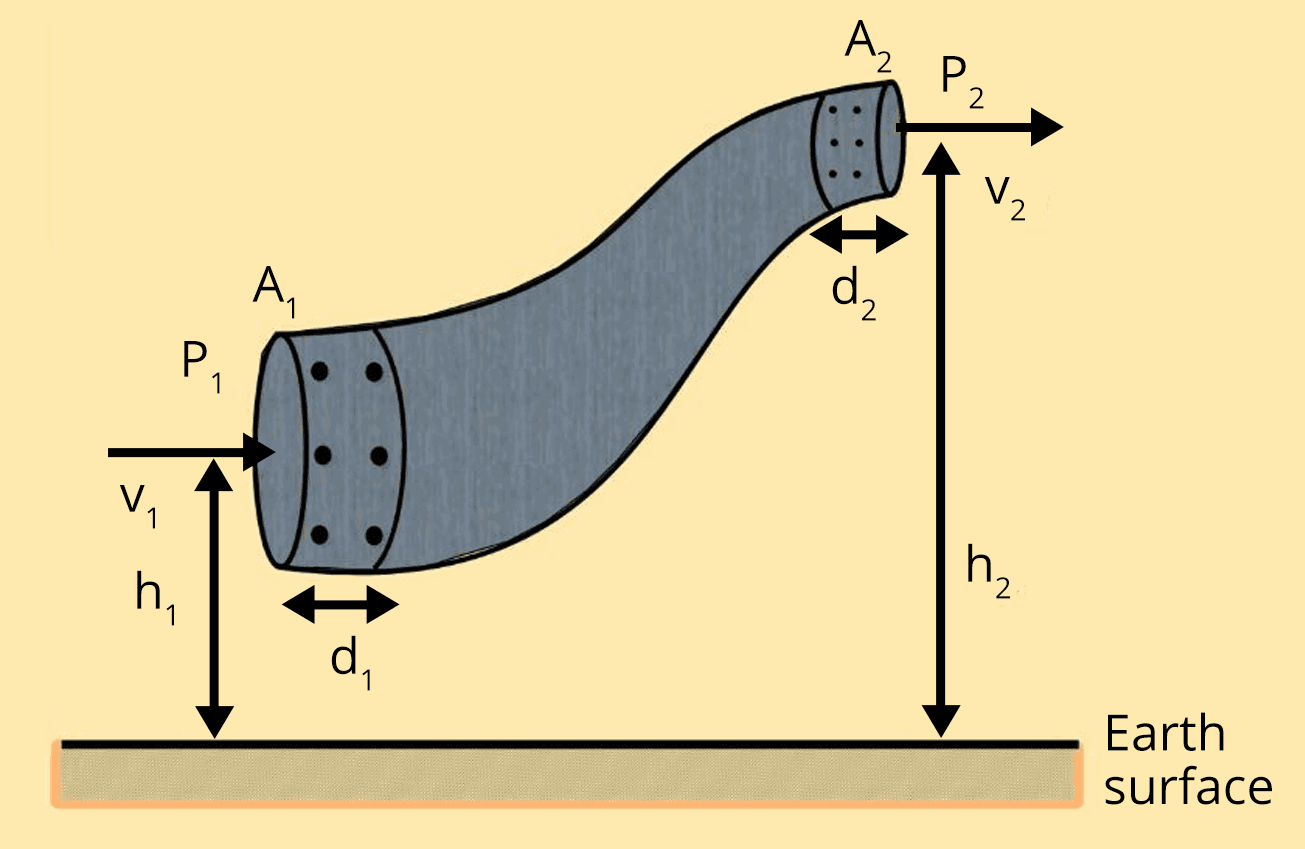$P_1+\rho gh_1+\dfrac{1}{2}\rho v_1^2=P_2+\rho gh_2+\dfrac{1}{2}\rho v_2^2$ Application of Bernoulli’s theorem One of the application of bernouilli’s theorem is the aerodynamic lift.(Image will be uploaded soon)Since the total energy of the fluid remains constant, fluid flowing through upper part of the body will have low pressure compared to the high pressure in the fluid passing through lower side of the body.Due to pressure difference, there is a net force or uplift which helps the body to move up.Another application is when the ball is moving and spinning in air.The ball changes its direction due to pressure difference and this effect is called magnus effect. Stoke’s Law When a body falls through a fluid, it drags the layer of the fluid in contact with it, a motion between the different layers of the fluid is set and, as a result the body experiences a retarding force, which is known as Viscous Force.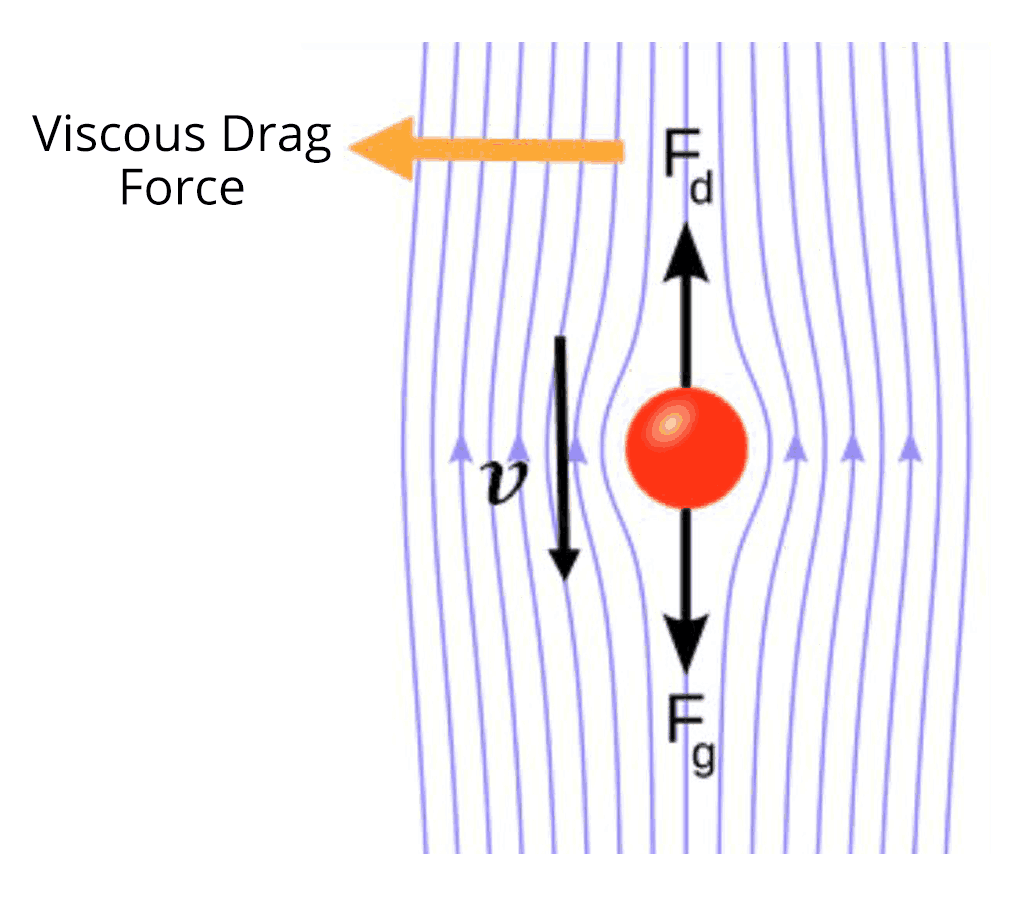$F=6\pi\eta rv$Where η is the coefficient of viscosity, r is the radius of the sphere and v is the velocity of the body.When a body is falling through a liquid, it attains a constant speed when the net force acting on the body becomes zero. This is called terminal velocity. Surface tension and surface energy Surface tension is net effect of cohesive forces in downward direction on any surface.(Image will be uploaded soon)The unit of surface tension is N/m.Surface energy is the potential energy contained by  the molecules of the liquid surface due to which they stay on the surface against inward force of attraction by other molecules.The magnitude of surface energy per unit area and surface tension are equal.The relationship between surface tension ans surface energy is given by,$\text{Surface tension}=\dfrac{\text{Surface energy}}{\text{Area}}$

### List of Important Formulae

 Sl. No. Name of the Concept Formula 1. Work done in stretching a strain Work done in stretching a wire having young’s modulus(Y) under a force (F) is given by the formula,$W=\dfrac{1}{2}\dfrac{YA\Delta l^2}{l}$ 2. Thermal stress on a rod fixed between two rigid supports. $\text{Thermal stress }=Y\alpha\Delta T$Where ⍺ is the coefficient of linear expansion and ΔT is the change in temperature 3. Force constant of the wire having a length(l) and area of cross section(A) $K=\dfrac{YA}{l}$ 4. Depression produced in the beam of bridge (Image will be uploaded soon)$\delta=\dfrac{Wl^3}{4Ybd^3}$ 5. Energy stored per unit volume of wire $U_V=\dfrac{1}{2}\times\text{stress}\times\text{strain}$$U_V=\dfrac{1}{2}\times Y\times\text{strain}^2$ 6. Variation Of Pressure With Depth Inside The Fluid $P_2-P_1=\rho gh$(Image will be uploaded soon) 7. Equation of continuity $A_1V_1=A_2V_2$Where A1 and A2 are the area of cross section of the pipe at the two ends and V1 and V2 are the corresponding velocity of the fluid.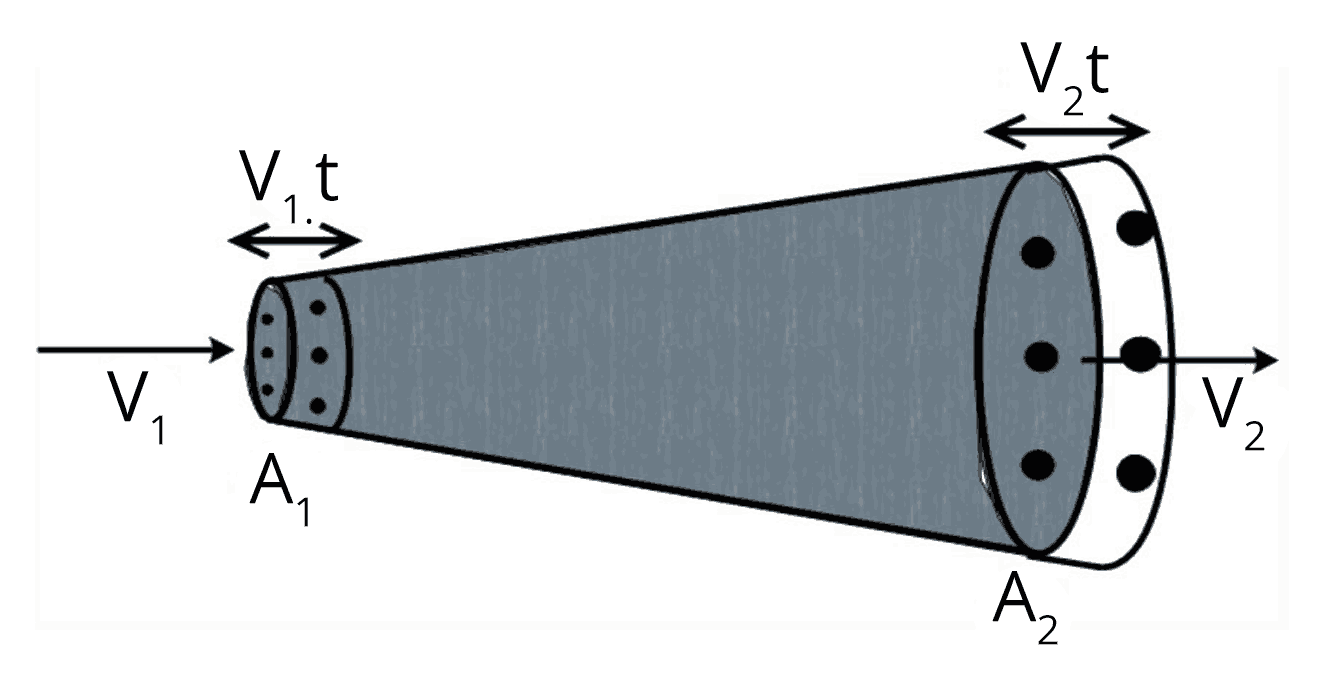8. Velocity of efflux Velcoity of water flowing out from the container is given by,$v=\sqrt{2gh}$(Image will be uploaded soon) 9. Excess pressure inside a liquid drop $p=\dfrac{2S}{r}$Where S is the surface tension and r is the radius of the drop 10. Excess pressure inside a liquid drop $p=\dfrac{4S}{r}$Where S is the surface tension and r is the radius of the bubble. 11. The height of capillary rise inside a tube $h=\dfrac{2S\cos\theta}{\rho rg}$(Image will be uploaded soon)

### Solved Examples

1. When a load of 5 kg is hung on a wire, then the extension of 3 m takes place. The work done will be,

Ans:

The mass of the load hung on the wire, m = 5 kg

The force acting on the wire is calculated as ,

$F=mg$

$F=5\times9.8$

$F=49~N$

We can calculate the work done in extending the wire by using the formula given below,

$W=\dfrac{1}{2}F\Delta l$

$W=\dfrac{1}{2}\times 49\times 3$

$W=73.5~J$

Key Point: The work done in extending the wire is half the product of the force acting on the wire and the extension of the wire.

1. In a capillary tube, water rises by 1.2 mm. The height of water will rise in another capillary tube having half the radius of the first will be ____.

Ans:

The formula to calculate the capillary rise of first tube is given by,

$h_1=\dfrac{2S\cos\theta}{\rho rg}$

$1.2~mm=\dfrac{2S\cos\theta}{\rho rg}$...(1)

The capillary rise of the water in the second tube is given by,

$h_2=\dfrac{2S\cos\theta}{\rho (r/2)g}$

$h_2=\dfrac{4S\cos\theta}{\rho rg}$.....(2)

Divide the equation (2) by equation (1) to calculate the height of capillary rise in second tube.

$\dfrac{h_2}{1.2~mm}=\dfrac{\dfrac{4S\cos\theta}{\rho rg}}{\dfrac{2S\cos\theta}{\rho rg}}$

$h_2=2\times2~mm$

$h_2=4~mm$

Key Point:  The surface tension and the angle of contact in both tubes are equal since the same liquid is used in both tubes.

### Previous Year Questions from JEE Exam

1. A hydraulic press can lift 100 kg when a mass ‘m’ is placed on the smaller piston. It can lift _______ kg when the diameter of the larger piston is increased by 4 times and that of the smaller piston is decreased by 4 times keeping the same mass ‘m’ on the smaller piston. (JEE 2021)

Ans:

Applying Pascal’s law, the pressure acting on smaller piston due to mass m is equal to the pressure acting on the larger piston.

Let A1 and A2 be the area of the smaller piston and larger piston respectively.

$\dfrac{mg}{A_1}=\dfrac{100g}{A_2}$

$\dfrac{A_1}{A_2}=\dfrac{m}{100}$...(1)

Now, let M be the mass that hydraulic press can lift after changing the area of cross section of both pistons.

Again applying pascal’s law,

$\dfrac{mg}{(A_1/16)}=\dfrac{Mg}{(16A_2)}$

$\dfrac{A_1}{A_2}=256\dfrac{m}{M}$...(2)

Using  equation (1) and equation (2), we can calculate the value of M.

$\dfrac{m}{100}=256\dfrac{m}{M}$

$M=25600~kg$

Therefore, the new mass it can lift is 25600 kg

Trick:  The pressure acting on both the piston are equal according to the Pascal’s law.

1. A uniform metallic wire is elongated by 0.04 m when subjected to a linear force F. The elongation, if its length and diameter is doubled and subjected to the same force will be ________ cm. (JEE 2021)

Ans:

The formula to calculate the young’s modulus of the wire is given by,

$Y=\dfrac{Fl}{A\Delta l_1}$....(1)

When the diameter is doubled, the area of cross section of the wire becomes four times initial area of cross section.

When the same force is applied after the length and diameter is doubled, then formula for young’s modulus is given by,

$Y=\dfrac{F(2l)}{(4A)\Delta l_2}$

$Y=\dfrac{Fl}{2A\Delta l_2}$....(2)

Using equation (1) and (2),

$\dfrac{Fl}{A\Delta l_1}=\dfrac{Fl}{2A\Delta l_2}$

$\Delta l_2=\dfrac{\Delta l_1}{2}$

$\Delta l_2=\dfrac{0.04}{2}$

$\Delta l_2=0.02~m=2~cm$

Therefore, the new extension will be 2 cm.

Trick: Young’s modulus deos not depend on the dimensions on the wire and only depends onthe material.

### Practice Questions

1. An iceberg of density 900 kg/m3 is floating in water of density 1000 kg/m3. The percentage of the volume of ice cube outside the water is ? (Ans: 10 %)

2. A wind with speed 40 m/s blows parallel to the roof of a house. The area of the roof is 250 m2. Assuming that the pressure inside the house is atmospheric pressure, the force exerted by the wind on the roof will be (Ans: 2.4✕105 N)

### Conclusion

In this article, we discussed important topics and formulas related to Properties of solids and liquids from the JEE point of view. Students must make sure that they do not miss any of the above important topics to obtain a good score in the JEE Main exam.

## Important Related Links for JEE Main 2022-23

See More## JEE Main Important Dates

View all JEE Main Exam Dates
JEE Main 2023 January and April Session exam dates and revised schedule have been announced by the NTA. JEE Main 2023 January and April Session will now be conducted on 24-Jan-2023 to 31-Jan-2023 and 6-Apr-2023 to 12-Apr-2023, and the exam registration closes on 12-Jan-2023 and Apr-2023. You can check the complete schedule on our site. Furthermore, you can check JEE Main 2023 dates for application, admit card, exam, answer key, result, counselling, etc along with other relevant information.
See More
View all JEE Main Exam Dates## JEE Main Information

Application Form
Eligibility Criteria
Reservation Policy
Exam Centres
NTA has announced the JEE Main 2023 January session application form release date on the official website https://jeemain.nta.nic.in/. JEE Main 2023 January and April session Application Form is available on the official website for online registration. Besides JEE Main 2023 January and April session application form release date, learn about the application process, steps to fill the form, how to submit, exam date sheet etc online. Check our website for more details. April Session's details will be updated soon by NTA.## JEE Main Syllabus

View JEE Main Syllabus in Detail
It is crucial for the the engineering aspirants to know and download the JEE Main 2023 syllabus PDF for Maths, Physics and Chemistry. Check JEE Main 2023 syllabus here along with the best books and strategies to prepare for the entrance exam. Download the JEE Main 2023 syllabus consolidated as per the latest NTA guidelines from Vedantu for free.
See More
View JEE Main Syllabus in Detail## JEE Main 2023 Study Material

View all study material for JEE Main
JEE Main 2023 Study Materials: Strengthen your fundamentals with exhaustive JEE Main Study Materials. It covers the entire JEE Main syllabus, DPP, PYP with ample objective and subjective solved problems. Free download of JEE Main study material for Physics, Chemistry and Maths are available on our website so that students can gear up their preparation for JEE Main exam 2023 with Vedantu right on time.
See More
All
Mathematics
Physics
Chemistry
See All## JEE Main Question Papers

see all
Download JEE Main Question Papers & ​Answer Keys of 2022, 2021, 2020, 2019, 2018 and 2017 PDFs. JEE Main Question Paper are provided language-wise along with their answer keys. We also offer JEE Main Sample Question Papers with Answer Keys for Physics, Chemistry and Maths solved by our expert teachers on Vedantu. Downloading the JEE Main Sample Question Papers with solutions will help the engineering aspirants to score high marks in the JEE Main examinations.
See MoreView all JEE Main Important Books
In order to prepare for JEE Main 2023, candidates should know the list of important books i.e. RD Sharma Solutions, NCERT Solutions, RS Aggarwal Solutions, HC Verma books and RS Aggarwal Solutions. They will find the high quality readymade solutions of these books on Vedantu. These books will help them in order to prepare well for the JEE Main 2023 exam so that they can grab the top rank in the all India entrance exam.
See More
Maths
NCERT Book for Class 12 Maths
Physics
NCERT Book for Class 12 Physics
Chemistry
NCERT Book for Class 12 Chemistry
Physics
H. C. Verma Solutions
Maths
R. D. Sharma Solutions
Maths
R.S. Aggarwal Solutions
See All## JEE Main Mock Tests

View all mock tests
JEE Main 2023 free online mock test series for exam preparation are available on the Vedantu website for free download. Practising these mock test papers of Physics, Chemistry and Maths prepared by expert teachers at Vedantu will help you to boost your confidence to face the JEE Main 2023 examination without any worries. The JEE Main test series for Physics, Chemistry and Maths that is based on the latest syllabus of JEE Main and also the Previous Year Question Papers.
See More## JEE Main 2023 Cut-Off

JEE Main Cut Off
NTA is responsible for the release of the JEE Main 2023 January and April Session cut off score. The qualifying percentile score might remain the same for different categories. According to the latest trends, the expected cut off mark for JEE Main 2023 January and April Session is 50% for general category candidates, 45% for physically challenged candidates, and 40% for candidates from reserved categories. For the general category, JEE Main qualifying marks for 2021 ranged from 87.8992241 for general-category, while for OBC/SC/ST categories, they ranged from 68.0234447 for OBC, 46.8825338 for SC and 34.6728999 for ST category.
See More## JEE Main 2023 Results

NTA will release the JEE Main 2023 January and April sessions exam dates on the official website, i.e. {official-website}. Candidates can directly check the date sheet on the official website or https://jeemain.nta.nic.in/. JEE Main 2023 January and April sessions is expected to be held in February and May. Visit our website to keep updates of the respective important events of the national entrance exam.
See More
Rank List
Counselling
Cutoff
JEE Main 2023 state rank lists will be released by the state counselling committees for admissions to the 85% state quota and to all seats in IIT colleges. JEE Main 2023 state rank lists are based on the marks obtained in entrance exams. Candidates can check the JEE Main 2023 state rank list on the official website or on our site.## JEE Top Colleges

View all JEE Main 2023 Top Colleges
Want to know which Engineering colleges in India accept the JEE Main 2023 scores for admission to Engineering? Find the list of Engineering colleges accepting JEE Main scores in India, compiled by Vedantu. There are 1622 Colleges that are accepting JEE Main. Also find more details on Fees, Ranking, Admission, and Placement.
See More## FAQs on JEE Important Chapter - Properties of Solids and Liquids

FAQ

1. How many questions are asked from properties os solids and liquids in JEE?

About 2-3 questions from this chapter are asked in JEE exam every year which corresponds to around 8-12 marks in the JEE Main exam.

2. Is the chapter properties of solids and liquids a tough chapter?

As far as teh JEE main exam is concerned, properties of solids and liquids is a moderate difficult chapter. But it is a vast chapte which consists of more theories and concepts and related formulas. But with proper learning and practices, all the concepts in this chapter can be learned without any difficulty.

3. How to get a good score in JEE Main to get into the best NITs?

To get into best NITs in the nation, we have to crack the JEE Main exam with good scores. Even though JEE Main is a very competitive exam, we can score good marks if we understand the concepts in depth and pratice lots of questions and finally doing all the previous year question papers without any fail.## Notice board

JEE News
JEE BlogsTrending pages